#### You may also like### Triangle Incircle Iteration

Keep constructing triangles in the incircle of the previous triangle. What happens?### Loopy

Investigate sequences given by $a_n = \frac{1+a_{n-1}}{a_{n-2}}$ for different choices of the first two terms. Make a conjecture about the behaviour of these sequences. Can you prove your conjecture?### Converging Means

Take any two positive numbers. Calculate the arithmetic and geometric means. Repeat the calculations to generate a sequence of arithmetic means and geometric means. Make a note of what happens to the two sequences.

# Stretching Fractions

##### Age 14 to 16Challenge Level

This problem is about an iterative process.

To iterate means to repeat, so an iterative process involves repeating something many times.

Imagine some dough, Plasticine or Blu-Tack, something that can be made into a strip then stretched.

We are going to take a length of this material, which we'll regard as the unit length, and put a mark at some fraction distance along it.

Now we are going to follow a procedure and see where our mark ends up.

1. The material is folded in the middle so that the bottom reaches back to the top.

2. The material is now only half a unit in length and twice as fat, so it is rolled out or stretched uniformly to become one unit in length again.

3. Finally we'll note the new position of our mark.

And that's the process we'll be repeating.

Now let's try with an actual fraction.

Starting for example at $\frac{1}{5}$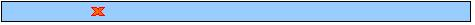First Iteration : We fold to get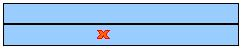then roll out, back to unit length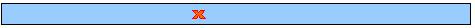The new position is $\frac{2}{5}$

Second Iteration : fold again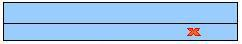and roll back out to unit length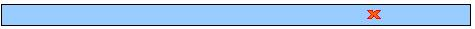The position is now $\frac{4}{5}$

Third Iteration : fold again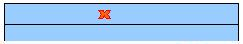and roll back out to unit lengthThe position is now $\frac{2}{5}$ for the second time

But we know what happens after $\frac{2}{5}$, it goes to $\frac{4}{5}$, then $\frac{2}{5}$ again, and so on for ever.

So what are you invited to investigate?
1. What happens for other start fractions?

2. Does everything go to a loop?

3. What size loops appear and for what fractions?

In fact you'll want to describe those loops very carefully.

This problem came to our attention via an ATM workshop led by Dave Hewitt, from the School of Education, University of Birmingham.
We appreciate his permission to pass it on.